# Introduction

So far, we have explained how to set up the RAPIDS functionality to use GPU for machine learning. In this article, we will walk you through the actual power of utilizing RAPIDS in comparison to Sklearn, along with their performance assessment with the Regression use case. But before diving in, let’s clear out some things that you have to know about the use case.

# About the Use Case & Data

The use case is the price prediction of the used cars obtained from the UCI Machine Learning Repository. The original data is less in size. So, to show the functionality of RAPIDS in comparison to Sklearn, we have synthesized it using Synthetic Data Vault (SDV) library, which you can download from here. If you want to download the cleaned version of the data, click here.

To load the data, we will use be using the pandas read_csv() method.

`path = “https://github.com/insaid2018/articles/blob/main/data/01-used_cars.csv"data = pd.read_csv(filepath_or_buffer=path)print(‘Data Shape:’, data.shape)Output:Data Shape: (100000, 27)`

# Data Cleaning

Next, we will clean/handle the data to prepare it for our model development. Please note that we have already identified the steps that will take place to clean the data. You may go ahead and analyze the data before taking any action.

`# Replacing ? with null valuesdata.replace(“?”, np.nan, inplace = True)# Estimating median values for missing featuresmedian_normalizedlosses = data[‘normalized-losses’].median()median_bore = data[‘bore’].median()median_stroke = data[‘stroke’].median()median_horsepower = data[‘horsepower’].median()median_peakrpm = data[‘peak-rpm’].median()mode_numofdoors = data[‘num-of-doors’].mode()# Imputing missing values with median value of featuresdata[‘normalized-losses’] = data[‘normalized-losses’].replace(np.nan, median_normalizedlosses)data[‘bore’] = data[‘bore’].replace(np.nan, median_bore)data[‘stroke’] = data[‘stroke’].replace(np.nan, median_stroke)data[‘horsepower’] = data[‘horsepower’].replace(np.nan, median_horsepower)data[‘peak-rpm’] = data[‘peak-rpm’].replace(np.nan, median_peakrpm)data[‘num-of-doors’] = data[‘num-of-doors’].replace(np.nan, mode_numofdoors)# Dropping the entire rows of missing price valuedata.dropna(subset=[‘price’], axis=0, inplace=True)# Converting features having inconsistent data types to consistent onedata[‘normalized-losses’] = data[‘normalized-losses’].astype(int)data[‘bore’] = data[‘bore’].astype(float)data[‘stroke’] = data[‘stroke’].astype(float)data[‘horsepower’] = data[‘horsepower’].astype(float)data[‘peak-rpm’] = data[‘peak-rpm’].astype(float)data[‘price’] = data[‘price’].astype(float)print(‘Success!’)Output:Success!`

# Feature Encoding

In this step, we will be encoding all the categorical features to numeric and then proceed with data scaling. Here, we will be using an advanced algorithm to encode the data known by the name of K-Fold Target Encoding.

`class KFoldTargetEncoder(BaseEstimator, TransformerMixin):    def __init__(self ,colnames , targetName, n_fold=5, verbosity=True, discardOriginal_col=False):        self.colnames = colnames        self.targetName = targetName        self.n_fold = n_fold        self.verbosity = verbosity        self.discardOriginal_col = discardOriginal_col     def fit(self, X, y=None):        return self    def transform(self, X):        assert(type(self.targetName) == str)        assert(type(self.colnames) == str)        assert(self.colnames in X.columns)        assert(self.targetName in X.columns)        mean_of_target = X[self.targetName].mean()        kf = KFold(n_splits = self.n_fold, shuffle = False, random_state = None)        col_mean_name = 'E_' + self.colnames        X[col_mean_name] = np.nan        for tr_ind, val_ind in kf.split(X):            X_tr, X_val = X.iloc[tr_ind], X.iloc[val_ind]            X.loc[X.index[val_ind], col_mean_name] =         X_val[self.colnames].map(X_tr.groupby(self.colnames)[self.targetName].mean())            X[col_mean_name].fillna(mean_of_target, inplace = True)         if self.verbosity:            encoded_feature = X[col_mean_name].values            print('Correlation between [{}] and, [{}] is {}.'.format(col_mean_name, self.targetName, np.corrcoef(X[self.targetName].values, encoded_feature)))        if self.discardOriginal_col:           X = X.drop(self.colnames, axis=1)        return Xcolumns = [‘make’, ‘fuel-type’, ‘aspiration’, ‘num-of-doors’,           ‘body-style’, ‘drive-wheels’, ‘engine-location’,           ‘engine-type’, ‘num-of-cylinders’, ‘fuel-system’]for column in columns:    k_object = KFoldTargetEncoder(colnames=column,                                  targetName=’price’,                                  discardOriginal_col=True)    data = kfold_make.fit_transform(X=data)encodedData = data`

# Feature Scaling

In this step, we will scale our data and split the data into input and target format so that we can proceed with the feature selection part.

`cols = encodedData.columns.to_list()price_index = cols.index(‘price’)print(‘Location of Price feature in encoded dataframe is ‘, price_index)# Creating scalers for each featurescalers = [MinMaxScaler() for obj in range(len(cols))]for scaler, col in zip(scalers, cols):    encodedData[col] = scaler.fit_transform(encodedData[[col]])normalizedData = encodedData`

# Feature Selection

Now that we have all the features on one scale, we will use an algorithmic approach to find essential data features for model development. In this step, we will be using a random forest model to get a subset of features (best) for model development.

`X = normalizedData.drop(labels=’price’, axis=1)y = normalizedData[‘price’]# Have some patience, may take some time :)selector = SelectFromModel(RandomForestRegressor(n_estimators = 100, random_state = 42, n_jobs = -1))selector.fit(X, y)# Extracting list of important featuresselected_feat = X.columns[(selector.get_support())].tolist()print(‘Total Features Selected are’, len(selected_feat))# Estimated by taking mean (default) of feature importanceprint(‘Threshold set by Model:’, np.round(selector.threshold_, decimals=2))print(‘Features:’, selected_feat)Output:Total Features Selected are 13 Threshold set by Model: 0.04 Features: ['ID', 'normalized-losses', 'wheel-base', 'length', 'width', 'height', 'curb-weight', 'engine-size', 'bore', 'stroke', 'compression-ratio', 'horsepower', 'E_make']`

# Data Splitting

Now, we will take only those features into account which are identified as best for model development by the random forest.

`# Get only essential featuresX = normalizedData[selected_feat]y = normalizedData[‘price’]# Split the data based on the essential features for model developmentX_train, X_test, y_train, y_test = train_test_split(X, y, test_size=0.1, random_state=42)# Display splitted data shapeprint(‘Training Data Shape:’, X_train.shape, y_train.shape)print(‘Testing Data Shape:’, X_test.shape, y_test.shape)Output:Training Data Shape: (89036, 12) (89036,) Testing Data Shape: (9893, 12) (9893,)`

# Model Development

In this step, we will develop machine models using Sklearn and RAPIDS and train them using GPUs. Please make sure that GPUs are running in your environment.

Model Development using Sklearn Algorithms

`sk_results = list()models = [skLinearRegression(), skRidge(random_state=42),          skLasso(random_state=42), skElasticNet(random_state=42),          skRandomForestRegressor(random_state=42),          skKNeighborsRegressor()]for model in models:    start_time = time.time()    model.fit(X_train, y_train)    duration = time.time() — start_time    y_train_pred = model.predict(X_train)    y_test_pred = model.predict(X_test)    rmse_train = np.round(np.sqrt(mean_squared_error(y_true=y_test, y_pred=y_test_pred)), decimals=2)    rmse_test = np.round(np.sqrt(mean_squared_error(y_true=y_test, y_pred=y_test_pred)), decimals=2)    r_squared_train = np.round(model.score(X_train, y_train), decimals=2)    r_squared_test = np.round(model.score(X_test, y_test), decimals=2)    record = [type(model).__name__, duration, rmse_train, rmse_test, r_squared_train, r_squared_test]    sk_results.append(record)    print(type(model).__name__, ‘Finished Training!’)Output:LinearRegression Finished Training! Ridge Finished Training! Lasso Finished Training! ElasticNet Finished Training! RandomForestRegressor Finished Training! KNeighborsRegressor Finished Training!`

Model Development using RAPIDS Algorithms

`cu_results = list()models = [cuLinearRegression(algorithm=’svd’), cuRidge(),          cuLasso(), cuElasticNet(),          cuRandomForestRegressor(random_state=42),          cuKNeighborsRegressor()]for model in models:    start_time = time.time()    model.fit(X_train, y_train)    duration = time.time() — start_time    rmse_train = np.round(np.sqrt(mean_squared_error(y_true=y_test, y_pred=y_test_pred)), decimals=2)    rmse_test = np.round(np.sqrt(mean_squared_error(y_true=y_test, y_pred=y_test_pred)), decimals=2)    r_squared_train = np.round(model.score(X_train, y_train), decimals=2) * 100    r_squared_test = np.round(model.score(X_test, y_test), decimals=2) * 100    record = [type(model).__name__, duration, rmse_train, rmse_test, r_squared_train, r_squared_test]    cu_results.append(record)    print(type(model).__name__, ‘Finished Training!’)LinearRegression Finished Training! Ridge Finished Training! Lasso Finished Training! ElasticNet Finished Training! RandomForestRegressor Finished Training! KNeighborsRegressor Finished Training!`

# Performance Analysis

Now that we have developed models using both Sklearn and RAPIDS libraries, it’s time to visualize their execution performance using the help of Plotly go. We have shown the performance using all the algorithms vs excluding random forest because it will be hard to visualize the clear difference. But before that, we need to create data frames of the results.

`columns = [‘Model (Sklearn)’, ‘Execution Time’, ‘RMSE (Train)’, ‘RMSE (Test)’, ‘R² (Train)’, ‘R² (Test)’]sk_frame = pd.DataFrame(data=sk_results, columns=columns)columns = ['Model (RAPIDS)', 'Execution Time', 'RMSE (Train)', 'RMSE (Test)', 'R^2 (Train)', 'R^2 (Test)']cu_frame = pd.DataFrame(data=cu_results, columns=columns)`

Let’s plot the graph.

`# Saving result excluding random forestsk_exclude_forest = sk_frame[sk_frame[‘Model (Sklearn)’] != ‘RandomForestRegressor’]cu_exclude_forest = cu_frame[cu_frame[‘Model (RAPIDS)’] != ‘RandomForestRegressor’]fig = go.Figure()fig = make_subplots(rows=2, cols=1, subplot_titles=[‘Execution Performance (All Models)’, ‘Execution Performance (Excluding Random Forest)’])fig.add_trace(go.Scatter(x=sk_frame[‘Model (Sklearn)’],                         y=sk_frame[‘Execution Time’]*1000,                         mode=’lines+markers’,                         name=’Sklearn’), row=1, col=1)fig.add_trace(go.Scatter(x=cu_frame[‘Model (RAPIDS)’],                         y=cu_frame[‘Execution Time’]*1000,                         mode=’lines+markers’,                         name=’RAPIDS’), row=1, col=1)fig.add_trace(go.Scatter(x=sk_exclude_forest[‘Model (Sklearn)’],                         y=sk_exclude_forest[‘Execution Time’]*1000,                         mode=’lines+markers’,                         name=’Sklearn’), row=2, col=1)fig.add_trace(go.Scatter(x=cu_exclude_forest[‘Model (RAPIDS)’],                         y=cu_exclude_forest[‘Execution Time’]*1000,                         mode=’lines+markers’,                         name=’RAPIDS’), row=2, col=1)# Updating figure layoutfig.update_layout(height=600, width=1200)# Updating axis labelsfig['layout']['xaxis']['title'] = 'Models'fig['layout']['xaxis2']['title'] = 'Models'fig['layout']['yaxis']['title'] = 'Execution Time (in milliseconds)'fig['layout']['yaxis2']['title'] = 'Execution Time (in milliseconds)'# Display the figurefig.show()`

And that’s it. We can see that RAPIDS is the new game in the market, and it is better than using Sklearn algorithms when it comes to using GPUs.

# What’s Next?

Classification in RAPIDS vs Sklearn

--

--

--

One of India’s leading institutions providing world-class Data Science & AI programs for working professionals with a mission to groom Data leaders of tomorrow!

Love podcasts or audiobooks? Learn on the go with our new app.

## Deep Learning can solve differential equations (theory & pytorch implementation)## Combining base learners of different types into a single meta-learner in an simple & intuitive way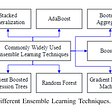## How VOTing classifiers work!## Performing Linear Regression in MicroPython-Raspberry Pi Pico (RP-2040)## Different evaluation metrics for regression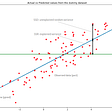## Probability and Statistics Notes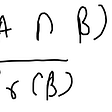## End to End ML pipelines with MLflow Projects## Filters as an image processing tool — part 1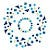## INSAID

One of India’s leading institutions providing world-class Data Science & AI programs for working professionals with a mission to groom Data leaders of tomorrow!

## Hyperparameter tuning/Tracking ML models using MLFLOW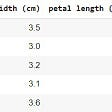## Train & Deploy, easy with AWS SageMaker## Cluster Analysis-Unsupervised ML for Pairs Trading using Indian Stock data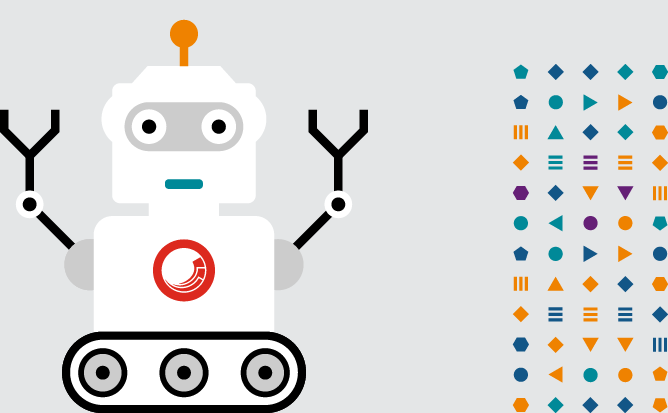## Extreme Gradient Boosting with XGBoost